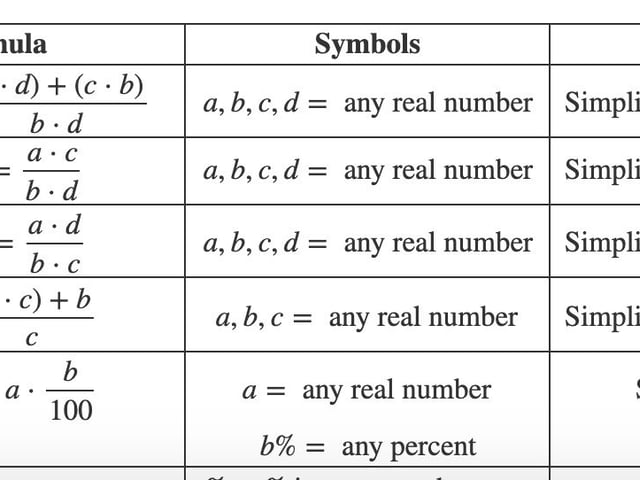# Helpful Formulas for the Accuplacer Next Generation Arithmetic Test

## Understanding the ACCUPLACER Arithmetic Next Generation Test

The ACCUPLACER Arithmetic Next Generation Test is a computer-adaptive test designed to evaluate a student’s understanding and ability to perform fundamental arithmetic operations. This test is commonly used by colleges and universities to assess incoming students’ readiness for college-level math courses, to identify students who may need additional support, or to place students in the correct math courses.

The ACCUPLACER Next Generation Test has 3 math-related sections: Next Generation Arithmetic; Next Generation Quantitative Reasoning, Algebra, and Statistics; and Next Generation Advanced Algebra and Functions.

## Test Structure

The Arithmetic test contains approximately 20 multiple-choice questions. These questions can be categorized into three broad content areas:

Whole number operations: This section tests basic arithmetic, including addition, subtraction, multiplication, division, and understanding number sequences.

Fraction operations: Here, the students’ ability to perform operations on fractions and mixed numbers are assessed. Questions might require addition, subtraction, multiplication, or division of fractions or conversion between improper fractions and mixed numbers.

Decimal and Percent operations: This section covers computations involving decimals and percents. It may involve conversion between decimals, fractions, and percent; addition, subtraction, multiplication, or division of decimals; and understanding percent increase or decrease.

## Preparation

Successful performance on the ACCUPLACER Arithmetic test requires a solid foundation in basic arithmetic concepts, including an understanding of number properties and types, as well as operations and their applications.

When preparing for this test, it’s crucial to review and understand the concepts of fractions, decimals, and percentages. You should also practice solving word problems, as these types of questions often appear on the test.

Finally, make sure to familiarize yourself with the test format and rules. Understanding the test’s structure and what is expected of you can reduce test anxiety and improve your performance.

The following chart gives you useful formulas that you’ll need to know if you want to ace the Arithmetic Section. While it’s not allowed to bring a formula chart into the test, understanding and memorizing key mathematical formulas will aid you during the exam. You won’t have access to a calculator, so you’ll need to rely on your basic arithmetic skills to succeed.

Check our ACCUPLACER Arithmetic study guides for more detailed information, and try to use these formulas for solving the practice tests we have at Union Test Prep!

## Fractions Formulas for the ACCUPLACER

Formula Symbols Comment
$$\dfrac{a}{b}+\dfrac{c}{d} = \dfrac{(a \cdot d)+(c \cdot b)}{b \cdot d}$$ $$a, b, c, d = \text{ any real number}$$ Simplify the fraction (if possible).
$$\dfrac{a}{b} \cdot \dfrac{c}{d} = \dfrac{a \cdot c}{b \cdot d}$$ $$a, b, c, d =\text{ any real number}$$ Simplify the fraction (if possible).
$$\dfrac{a}{b} \div \dfrac{c}{d} = \dfrac{a \cdot d}{b \cdot c}$$ $$a, b, c, d =\text{ any real number}$$ Simplify the fraction (if possible).
$$a \frac{b}{c} = \dfrac{(a\cdot c)+b}{c}$$ $$a, b, c=\text{ any real number}$$ Simplify the fraction (if possible).

## Percentage Formulas for the ACCUPLACER

Formula Symbols Comment
$$a \cdot b\% = a \cdot \dfrac{b}{100}$$ $$a = \text{ any real number}$$
$$b \%= \text{ any percent}$$
Simplify (if possible).
$$\%=\dfrac{\lvert b-a \rvert}{b} \cdot 100 = \dfrac{c}{b} \cdot 100$$ $$\% = \% \text{ increase or decrease}$$
$$a= \text{ new value}$$
$$b= \text{ original value}$$
$$c= \text{ amount of change}$$